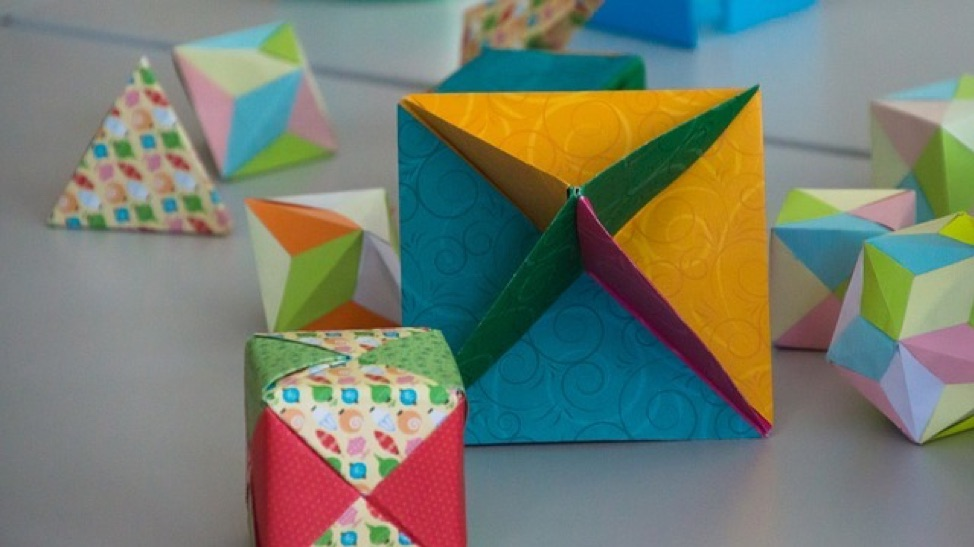# Conceptual Learning with MathBRIXImage by Taken from Pixabay

For many parents and educators, the shift to conceptual learning in mathematics was met with a mix of resistance, confusion, and frustration. It didn’t look or feel like math to those of us conditioned to drill, memorize, and sequence steps.

As the saying goes, though, time is the best teacher. Attitudes about this "new math" changed after countless teachers began implementing the conceptual math method. Seeing the results with their students, teachers felt encouraged and more positive about the approach. Now widely accepted and adopted, conceptual math is here to stay as an important component to effective pedagogy.

A growing number of tech-based tools, including MathBRIX, are available to support student competency in math by utilizing the research-based conceptual learning approach that leads to higher-level mathematical understanding.

## What is Conceptual Math?

Conceptual math is a method of math instruction that aims to increase literacy in mathematics rather than teach steps to find solutions. Often contrasted with procedural math instruction, conceptual math focuses on explaining why processes work rather than on how to perform a process.

A great example to illustrate a conceptual versus procedural approach is with subtraction that requires regrouping, or borrowing. If your elementary math education concluded before the 2010’s, chances are you learned how to borrow in order to solve some two (or more) digit math problems.

Let’s assume you were presented with the equation 54-29. It is likely that your teacher showed you steps to take: cross out the ones digit and change it to one less plus a ten (18 in this example) and then change the tens digit to one less (4 in this case). It is also likely that the reasoning behind that process was not explained or understood.

With the conceptual learning approach, students would have schema, or prior knowledge and experience to draw on, that would help them understand how numbers are composed and can be broken apart.

In our example, 54 can be broken down to (50+4) and 29 can also be seen as (20+9). 54-29, therefore, can be expressed as (50+4) - (20+9) and then (40+14) - (20+9). This gives context to borrowing and a much deeper understanding of mathematics than merely memorizing a sequence of steps.

It builds mathematical literacy.

Conceptual math understanding is not something that comes later in the education of a child, either. It begins in early childhood with the use of manipulatives and visual math, where pictures represent numbers, patterns, and equations and young children begin solving problems based on those visuals or manipulatives.Image by Klimkin from Pixabay

## What the Research Says About Conceptual Math

Many national groups have researched the efficacy and importance of using conceptual math in the classroom. Reports from the National Council of Teachers of Mathematics, the National Science Board, and the National Assessment of Educational Progress all conclude the following:

• The focus of math in school should be problem solving.
• The study of mathematics should emphasize higher order thinking skills, deep understanding of concepts, mathematical communication, making connections, and applying mathematics to other areas of learning.
• Basic skills in mathematics should go well beyond just computational ability and should be integrated to include things like logic, numeration, patterns, and relations.
• Mathematics programs should take advantage of available technology.

## The Benefits of Conceptual Learning in Math

When students only learn procedures, they often find themselves stuck and confused in higher level math courses. Conceptual math aims to help students understand why the steps work so that they can approach problem solving with a variety of strategies. Further, conceptual math provides a means to communicate mathematical ideas, and the ability to transfer that working knowledge to higher levels of problem solving and to other fields of study, such as science or engineering.

This is not to say that procedural math is not an important part of the overall picture. In fact, it is integral, working together with conceptual learning to move a student from concrete to abstract understanding. Mathematical competency relies on both conceptual and procedural competency.

## How MathBRIX Uses Conceptual Learning to Teach Math

MathBRIX allows students to use visual math and digital manipulatives to acquire new skills, problem solve, and practice curriculum. Using a “trunk and leaves” approach, students progress from concrete ideas to more abstract ones as their conceptual understanding increases.

Levels within MathBRIX are aligned with Common Core, the National Council of Teachers of Mathematics, and the Texas Essential Knowledge and Skills (TEKS) standards and each standard has a large variety of corresponding games. This gives students the opportunity to practice the same core competency in a multitude of ways, coming up with new ways to solve similar problems. This ability to utilize multiple approaches to solve a single problem is one of the pillars of conceptual learning.

MathBRIX uses language and visuals derived from the federally-funded Comprehensive School Mathematics Program (CSMP) and provides practice with newer curricula such as Singapore Math, Engage NY, Math in Focus, and Eureka Math, all of which follow the proven method of conceptual math.

Utilizing technology readily available in most modern elementary classrooms, MathBRIX is easy for students to use and targeted to individual learning needs. Teachers are well informed of progress, time on task, and achievement with MathBRIX’s unmatched tracking and reporting dashboard.

Used 15 minutes a day a couple of times a week, MathBRIX is a fun, game-based program rooted firmly in conceptual learning that makes an ideal supplement to any classroom serving students of all abilities aged 4-8 years old.